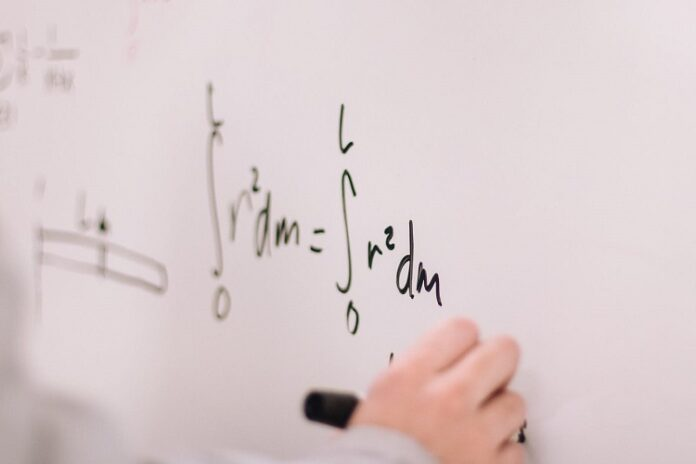# What Are Equivalent Ratios, And Why Are They Important In Math?

0
63Ratios are a fundamental concept in mathematics, and they are used to compare two or more quantities in a simplified form. Ratios are expressed as a fraction or a colon, and they represent the relationship between the quantities being compared. One type of ratio that is particularly important in math is equivalent ratios. Equivalent ratios have the same relationship between quantities as other ratios, but they are expressed in different forms. For example, the ratios 1:2 and 2:4 are equivalent because they represent the same relationship between the quantities, but they are expressed differently. Equivalent ratios are crucial in many math problems, especially those related to proportion, scaling, and probability. Understanding how to work with equivalent ratios is essential for solving these problems effectively. In this blog post, we will explore what ratios and equivalent ratios are, why they are important in math, and how to use them in various mathematical applications.

## What are Equivalent Ratios?

Equivalent ratios are two or more ratios with the same value or proportion. They represent the exact relationship between two or more numbers but are expressed differently. In other words, these are multiple fractions that look different but represent the same value.

For instance, consider the following two ratios:

• 4/6
• 8/12

At first glance, they may seem different. However, they are equal because they represent the exact value of 2/3. To determine if two ratios are equivalent, you can cross-multiply and simplify them; if they have the same value, they are equal.

## Why are Equivalent Ratios Important in Math?

Below are some of the essential applications of ratios in mathematics:

### Simplifying Fractions

When you have a fraction, not in its simplest form, you can find its equivalent simplest form by dividing the numerator and the denominator by their greatest common factor. This process reduces the fraction to its lowest terms.

For instance, consider the fraction 24/36. You can simplify this fraction by finding an equivalent ratio in its simplest form. To do this, you can find the greatest common factor (GCF) of 24 and 36, 12. Then you can divide the numerator and denominator by 12, which will be your 2/3 in its simplest form.

### Comparing Proportions

Another critical application of proportions is in comparing proportions. You can determine which is greater when you have two or more ratios by finding their equivalent proportion with the same denominator. Then, you can compare the numerators to see which one is greater.

For instance, consider the following two ratios:

• 5/8
• 3/5

You can find their equivalent ratios with the same denominator to compare these ratios. In this case, you can multiply the numerator and denominator of the first ratio by 5 and the numerator and denominator of the second ratio by 8, giving us the following:

• 25/40
• 24/40

Now, you can compare the numerators to determine which ratio is greater. In this case, the first ratio is greater than the second ratio.

### Solving Ratio Problems

In math, ratio problems involve comparing two or more quantities in proportion. For instance, if you have a recipe requiring 2 cups of flour for every 3 cups of sugar and want to make half the recipe, you must find the equivalent ratio representing half of the original recipe.

To do this, you can find the original recipe’s equal proportion by dividing the numerator and denominator by their greatest common factor. Then, you can multiply the resulting ratio by 1/2 to get the equivalent ratio representing half the recipe. In this case, the equal ratio representing half the recipe is 1 cup of flour for every 1.5 cups of sugar.

In conclusion, equivalent ratios are an essential concept in math that represents multiple fractions with the same value or proportion. They are necessary for simplifying fractions, comparing proportions, and solving ratio problems. By understanding equivalent ratios, learners can accurately compare numbers and solve complex math problems related to ratios. Thus, it is essential to have a solid understanding of ratios and proportions to excel in math and related fields.